moveit2 The MoveIt Motion Planning Framework for ROS 2.
moveit::core::Transforms Class Reference

Provides an implementation of a snapshot of a transform tree that can be easily queried for transforming different quantities. Transforms are maintained as a list of transforms to a particular frame. All stored transforms are considered fixed. More...

`#include <transforms.h>`

Inheritance diagram for moveit::core::Transforms: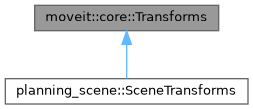[legend]

## Public Member Functions

Transforms (const Transforms &)=delete
Transforms cannot be copy-constructed. More...

Transformsoperator= (const Transforms &)=delete
Transforms cannot be copy-assigned. More...

Transforms (const std::string &target_frame)
Construct a transform list. More...

virtual ~Transforms ()
Destructor. More...

const std::string & getTargetFrame () const
Get the planning frame corresponding to this set of transforms. More...

virtual bool canTransform (const std::string &from_frame) const
Check whether data can be transformed from a particular frame. More...

virtual bool isFixedFrame (const std::string &frame) const
Check whether a frame stays constant as the state of the robot model changes. This is true for any transform mainatined by this object. More...

virtual const Eigen::Isometry3d & getTransform (const std::string &from_frame) const
Get transform for from_frame (w.r.t target frame) More...

Setting and retrieving transforms maintained in this class
const FixedTransformsMapgetAllTransforms () const
Return all the transforms. More...

void copyTransforms (std::vector< geometry_msgs::msg::TransformStamped > &transforms) const
Get a vector of all the transforms as ROS messages. More...

void setTransform (const Eigen::Isometry3d &t, const std::string &from_frame)
Set a transform in the transform tree (adding it if necessary) More...

void setTransform (const geometry_msgs::msg::TransformStamped &transform)
Set a transform in the transform tree (adding it if necessary) More...

void setTransforms (const std::vector< geometry_msgs::msg::TransformStamped > &transforms)
Set a transform in the transform tree (adding it if necessary) More...

void setAllTransforms (const FixedTransformsMap &transforms)
Set all the transforms: a map from string names of frames to corresponding Eigen::Isometry3d (w.r.t the planning frame) More...

Applying transforms
void transformVector3 (const std::string &from_frame, const Eigen::Vector3d &v_in, Eigen::Vector3d &v_out) const
Transform a vector in from_frame to the target_frame Note: assumes that v_in and v_out are "free" vectors, not points. More...

void transformQuaternion (const std::string &from_frame, const Eigen::Quaterniond &q_in, Eigen::Quaterniond &q_out) const
Transform a quaternion in from_frame to the target_frame. More...

void transformRotationMatrix (const std::string &from_frame, const Eigen::Matrix3d &m_in, Eigen::Matrix3d &m_out) const
Transform a rotation matrix in from_frame to the target_frame. More...

void transformPose (const std::string &from_frame, const Eigen::Isometry3d &t_in, Eigen::Isometry3d &t_out) const
Transform a pose in from_frame to the target_frame. More...

## Static Public Member Functions

static bool sameFrame (const std::string &frame1, const std::string &frame2)
Check if two frames end up being the same once the missing / are added as prefix (if they are missing) More...

## Protected Attributes

std::string target_frame_

FixedTransformsMap transforms_map_

## Detailed Description

Provides an implementation of a snapshot of a transform tree that can be easily queried for transforming different quantities. Transforms are maintained as a list of transforms to a particular frame. All stored transforms are considered fixed.

Definition at line 58 of file transforms.h.

## ◆ Transforms() [1/2]

 moveit::core::Transforms::Transforms ( const Transforms & )
delete

Transforms cannot be copy-constructed.

## ◆ Transforms() [2/2]

 moveit::core::Transforms::Transforms ( const std::string & target_frame )

Construct a transform list.

Definition at line 51 of file transforms.cpp.

## ◆ ~Transforms()

 moveit::core::Transforms::~Transforms ( )
virtualdefault

Destructor.

## ◆ canTransform()

 bool moveit::core::Transforms::canTransform ( const std::string & from_frame ) const
virtual

Check whether data can be transformed from a particular frame.

Reimplemented in planning_scene::SceneTransforms.

Definition at line 122 of file transforms.cpp.

## ◆ copyTransforms()

 void moveit::core::Transforms::copyTransforms ( std::vector< geometry_msgs::msg::TransformStamped > & transforms ) const

Get a vector of all the transforms as ROS messages.

Parameters
 transforms The output transforms. They are guaranteed to be valid isometries.

Definition at line 172 of file transforms.cpp.

Here is the caller graph for this function: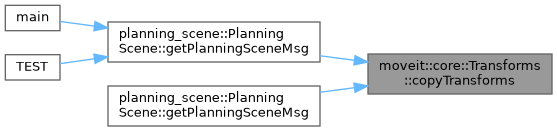## ◆ getAllTransforms()

 const FixedTransformsMap & moveit::core::Transforms::getAllTransforms ( ) const

Return all the transforms.

Returns
A map from string names of frames to corresponding Eigen::Isometry3d (w.r.t the planning frame). The transforms are guaranteed to be valid isometries.

Definition at line 78 of file transforms.cpp.

## ◆ getTargetFrame()

 const std::string & moveit::core::Transforms::getTargetFrame ( ) const

Get the planning frame corresponding to this set of transforms.

Returns
The planning frame

Definition at line 73 of file transforms.cpp.

Here is the caller graph for this function: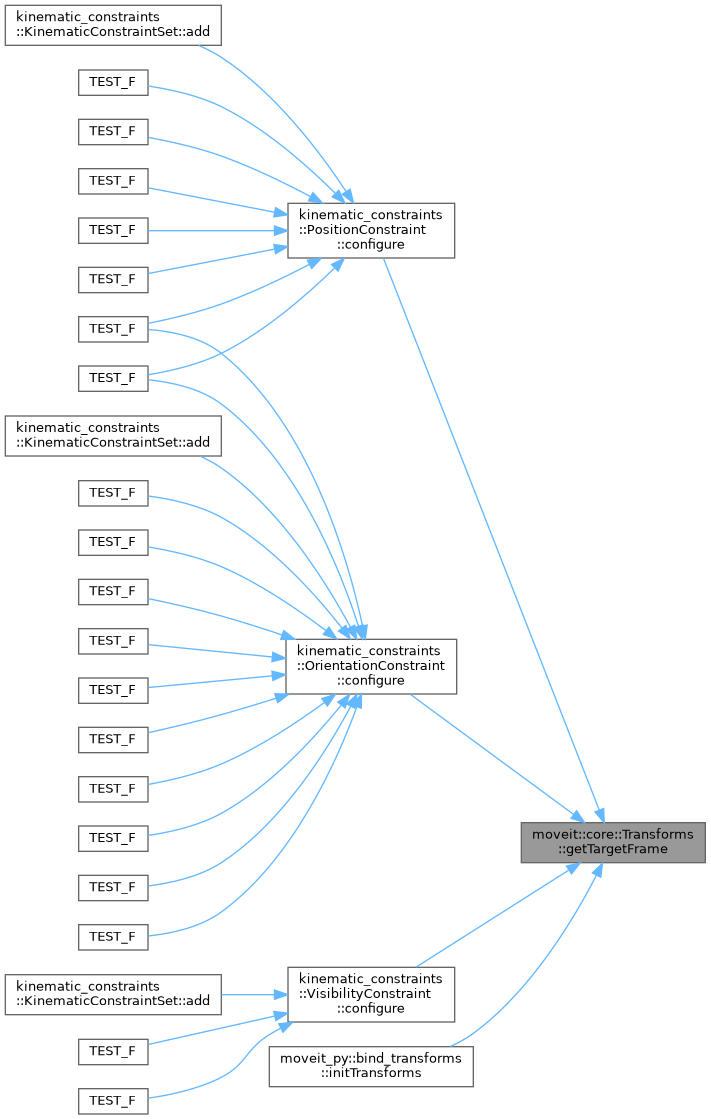## ◆ getTransform()

 const Eigen::Isometry3d & moveit::core::Transforms::getTransform ( const std::string & from_frame ) const
virtual

Get transform for from_frame (w.r.t target frame)

Parameters
 from_frame The string id of the frame for which the transform is being computed
Returns
The required transform. It is guaranteed to be a valid isometry.

Reimplemented in planning_scene::SceneTransforms.

Definition at line 104 of file transforms.cpp.

Here is the caller graph for this function: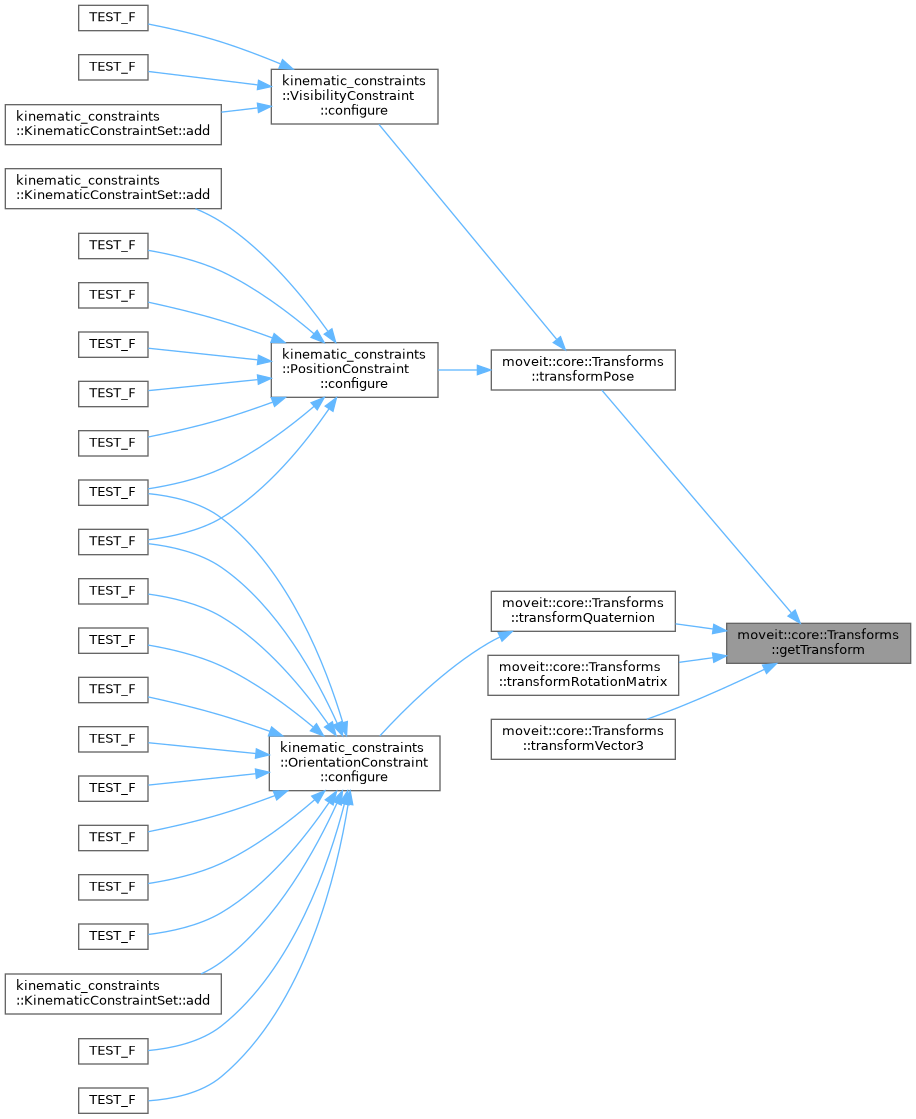## ◆ isFixedFrame()

 bool moveit::core::Transforms::isFixedFrame ( const std::string & frame ) const
virtual

Check whether a frame stays constant as the state of the robot model changes. This is true for any transform mainatined by this object.

Reimplemented in planning_scene::SceneTransforms.

Definition at line 92 of file transforms.cpp.

Here is the caller graph for this function: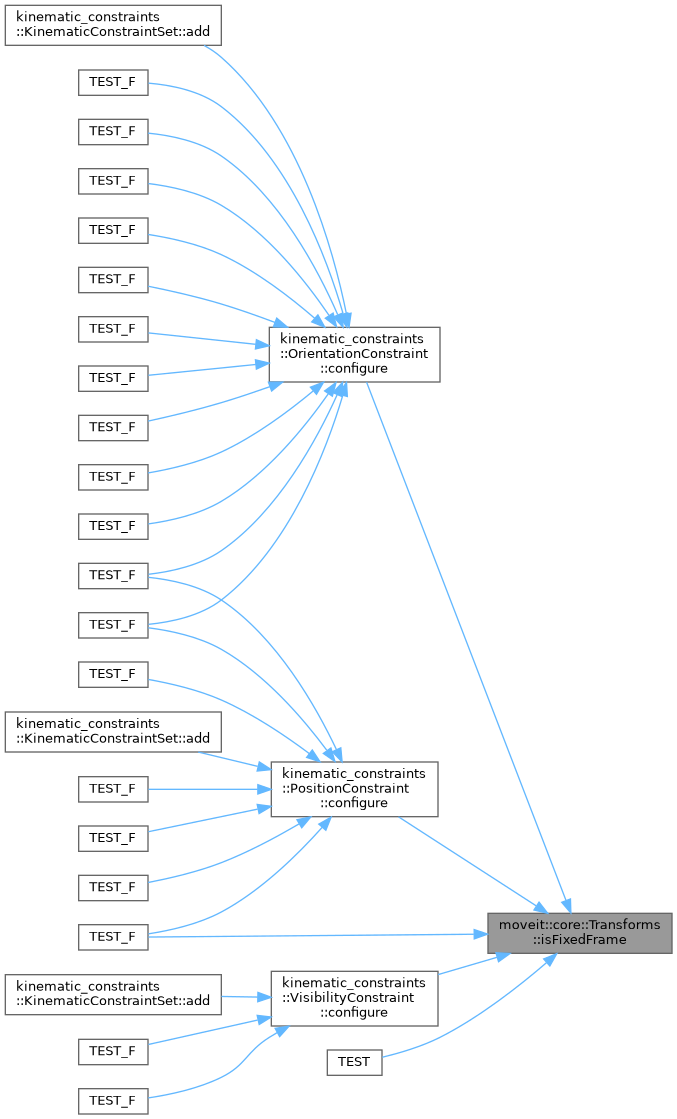## ◆ operator=()

 Transforms& moveit::core::Transforms::operator= ( const Transforms & )
delete

Transforms cannot be copy-assigned.

## ◆ sameFrame()

 bool moveit::core::Transforms::sameFrame ( const std::string & frame1, const std::string & frame2 )
static

Check if two frames end up being the same once the missing / are added as prefix (if they are missing)

Definition at line 64 of file transforms.cpp.

Here is the caller graph for this function: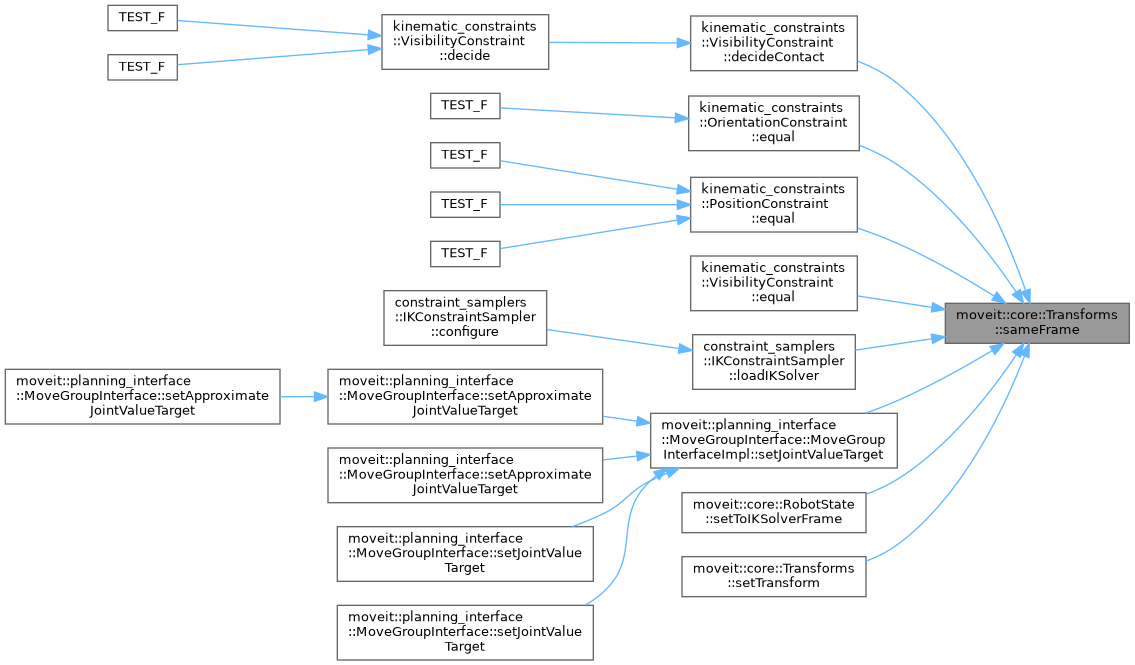## ◆ setAllTransforms()

 void moveit::core::Transforms::setAllTransforms ( const FixedTransformsMap & transforms )

Set all the transforms: a map from string names of frames to corresponding Eigen::Isometry3d (w.r.t the planning frame)

Definition at line 83 of file transforms.cpp.

## ◆ setTransform() [1/2]

 void moveit::core::Transforms::setTransform ( const Eigen::Isometry3d & t, const std::string & from_frame )

Set a transform in the transform tree (adding it if necessary)

Parameters
 t The input transform (w.r.t the target frame) from_frame The frame for which the input transform is specified

Definition at line 134 of file transforms.cpp.

Here is the caller graph for this function: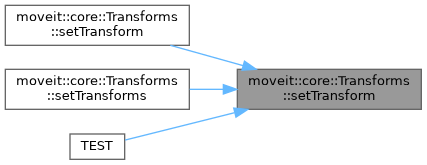## ◆ setTransform() [2/2]

 void moveit::core::Transforms::setTransform ( const geometry_msgs::msg::TransformStamped & transform )

Set a transform in the transform tree (adding it if necessary)

Parameters
 transform The input transform (the frame_id must match the target frame)

Definition at line 145 of file transforms.cpp.

Here is the call graph for this function: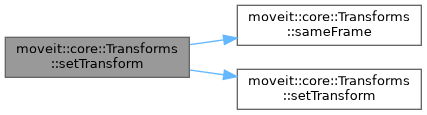## ◆ setTransforms()

 void moveit::core::Transforms::setTransforms ( const std::vector< geometry_msgs::msg::TransformStamped > & transforms )

Set a transform in the transform tree (adding it if necessary)

Parameters
 transform The input transforms (the frame_id must match the target frame)

Definition at line 166 of file transforms.cpp.

Here is the call graph for this function: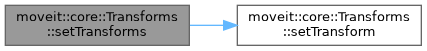## ◆ transformPose()

 void moveit::core::Transforms::transformPose ( const std::string & from_frame, const Eigen::Isometry3d & t_in, Eigen::Isometry3d & t_out ) const
inline

Transform a pose in from_frame to the target_frame.

Parameters
 from_frame The frame in which the input pose is specified t_in The input pose (in from_frame). Make sure the pose is a valid isometry. t_out The resultant (transformed) pose. It is guaranteed to be a valid isometry.

Definition at line 184 of file transforms.h.

Here is the call graph for this function: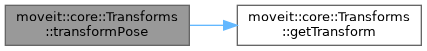Here is the caller graph for this function: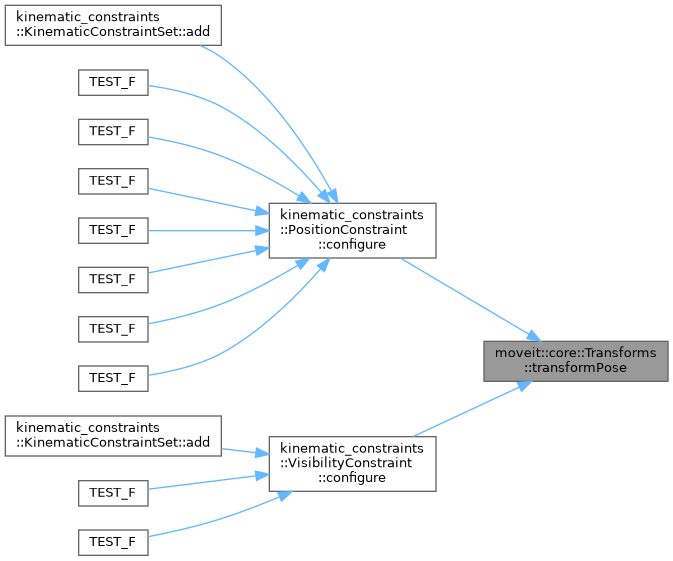## ◆ transformQuaternion()

 void moveit::core::Transforms::transformQuaternion ( const std::string & from_frame, const Eigen::Quaterniond & q_in, Eigen::Quaterniond & q_out ) const
inline

Transform a quaternion in from_frame to the target_frame.

Parameters
 from_frame The frame in which the input quaternion is specified v_in The input quaternion (in from_frame). Make sure the quaternion is normalized. v_out The resultant (transformed) quaternion. It is guaranteed to be a valid and normalized quaternion.

Definition at line 159 of file transforms.h.

Here is the call graph for this function: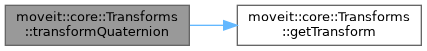Here is the caller graph for this function: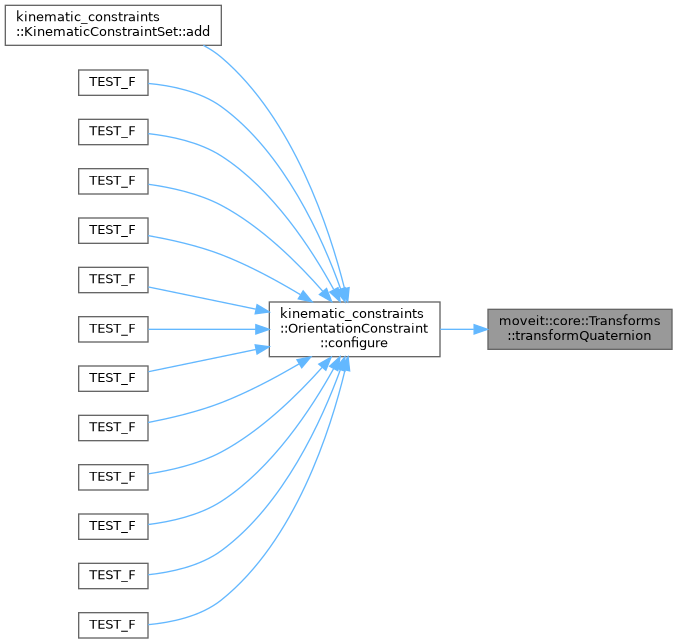## ◆ transformRotationMatrix()

 void moveit::core::Transforms::transformRotationMatrix ( const std::string & from_frame, const Eigen::Matrix3d & m_in, Eigen::Matrix3d & m_out ) const
inline

Transform a rotation matrix in from_frame to the target_frame.

Parameters
 from_frame The frame in which the input rotation matrix is specified m_in The input rotation matrix (in from_frame). Make sure the matrix is a valid rotation matrix. m_out The resultant (transformed) rotation matrix. It is guaranteed to be a valid rotation matrix.

Definition at line 172 of file transforms.h.

Here is the call graph for this function: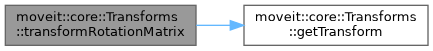## ◆ transformVector3()

 void moveit::core::Transforms::transformVector3 ( const std::string & from_frame, const Eigen::Vector3d & v_in, Eigen::Vector3d & v_out ) const
inline

Transform a vector in from_frame to the target_frame Note: assumes that v_in and v_out are "free" vectors, not points.

Parameters
 from_frame The frame from which the transform is computed v_in The input vector (in from_frame) v_out The resultant (transformed) vector

Definition at line 147 of file transforms.h.

Here is the call graph for this function: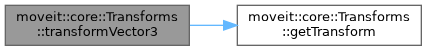## ◆ target_frame_

 std::string moveit::core::Transforms::target_frame_
protected

Definition at line 210 of file transforms.h.

## ◆ transforms_map_

 FixedTransformsMap moveit::core::Transforms::transforms_map_
protected

Definition at line 211 of file transforms.h.

The documentation for this class was generated from the following files: